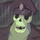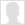Captain 26.08.2012 13:51 HERP, SWEET DERP

Man is spirit. But what is spirit? Spirit is the self. But what is the self? The self is a relation which relates itself to its own self, or it is that in the relation [which accounts for it] that the relation relates itself to its own self; the self is not the relation but [consists in the fact] that the relation relates itself to its own self. Man is a synthesis of the infinite and the finite, of the temporal and the eternal, of freedom and necessity, in short it is a synthesis. A synthesis is a relation between two factors. So regarded, man is not yet a self.
In the relation between two, the relation is the third term as a negative unity, and the two relate themselves to the relation, and in the relation to the relation; such a relation is that between soul and body, when man is regarded as soul. If on the contrary the relation relates itself to its own self, the relation is then the positive third term, and this is the self.1. rtsome 26.08.2012 17:39

The missile knows where it is at all times. It knows this because it knows where it isn't. By subtracting where it is from where it isn't, or where it isn't from where it is (whichever is greater), it obtains a difference, or deviation. The guidance subsystem uses deviations to generate corrective commands to drive the missile from a position where it is to a position where it isn't, and arriving at a position where it wasn't, it now is. Consequently, the position where it is, is now the position that it wasn't, and it follows that the position that it was, is now the position that it isn't.

In the event that the position that it is in is not the position that it wasn't, the system has acquired a variation, the variation being the difference between where the missile is, and where it wasn't. If variation is considered to be a significant factor, it too may be corrected by the GEA. However, the missile must also know where it was.

The missile guidance computer scenario works as follows. Because a variation has modified some of the information the missile has obtained, it is not sure just where it is. However, it is sure where it isn't, within reason, and it knows where it was. It now subtracts where it should be from where it wasn't, or vice-versa, and by differentiating this from the algebraic sum of where it shouldn't be, and where it was, it is able to obtain the deviation and its variation, which is called error.

Do you really want to delete ?